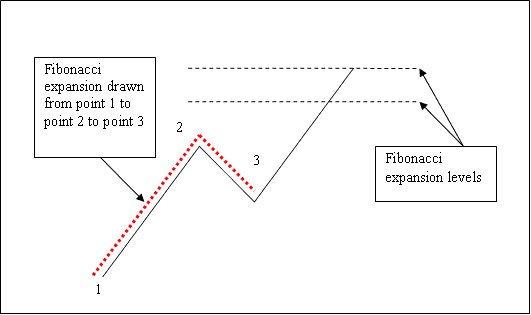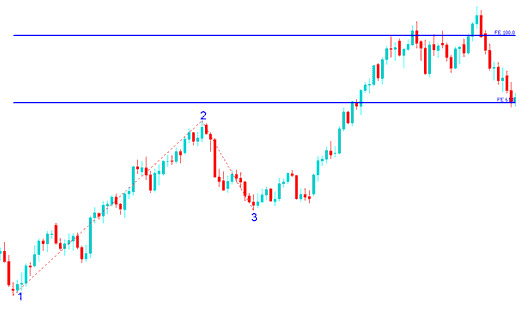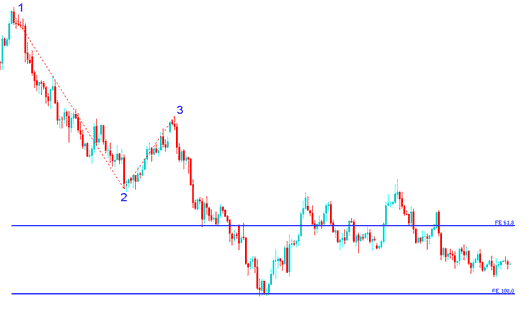# Drawing Fibonacci Expansion Levels on Upward and Downward Gold Trend

We have looked at Fibonacci retracement in the previous learn Gold trading lesson, drawn between 2 chart points. But to plot Fibonacci expansion levels we use 3 chart points.

To draw these levels we wait until the retracement is complete and the XAUUSD metal starts to move in the original direction of the Gold price trend. Where the retracement reaches is used as point 3.

The example below shows the 3 Points where the Fibonacci extension levels indicator is drawn - marked as 1, 2 and 3. Point 1 is where the trend started, Point 2 is where the trend started to be pulled back and retraced and Point 3 is where the retracement reached as shown on the example below.Fibonacci Expansion Levels

Please note where these Fibonacci expansion levels are plotted - they are drawn above the indicator, these are the points where the trader will set the take profit orders.

## Drawing Fibonacci Expansion Levels on an Upward Gold Price Trend

We use Fibonacci extension levels to estimate where the movement will reach. There are 2 important extension levels; 61.8 % Fibonacci expansion level and 100 % Fibonacci expansion level, these levels are used for taking profit.

On the example below you can see that the Fibonacci extension is plotted along the direction of the trend, since the trend is upward - the Fibonacci expansion is plotted upwards.

These Fibonacci expansion levels are displayed as horizontal lines above the indicator, showing profit taking areas. In the example below if you had used of 100 % extension you would have made nice profit from the buy trade.

NB: This is the same trade from the previous example where we used Fibonacci retracement to buy at around retracement level 38.2 %Fibonacci retracement level. At the same time we have used Fibonacci expansion level 100 % to set take profit. Now find a Gold price chart and practice these strategies.Fibonacci Expansion Levels Drawn on an Upward Gold Price Trend

From the above examples, the upward trend continued and both Fibonacci Expansion 61.8 % and 100.0 % levels were all hit after which price retraced again after hitting the 100.0 % Fibonacci extension level.

## Drawing Fibonacci Expansion Levels on a Downward Gold Price Trend

Since we use this tool to estimate take profits, how do we draw it in a downward Gold price trend?

We plot Fibonacci expansion levels indicator from point 1 to 2 to 3 as shown below. Remember we always plot this tool in the direction of the trend. In the example below, can you figure what direction we have drawn it? That is right - downwards because the trend is downwards.
Try and see the difference between how we have drawn it above and how it is drawn below. This time you would also have used Fibonacci extension level 100 %, see just where the price reached. That would have been a nice take profit area.Fibonacci Expansion Levels Drawn on a Downward Gold Price Trend

From the example above, after plotting this Fibonacci expansion tool there are two levels that are used to show the profit taking areas, these two levels are plotted as horizontal lines across the price chart (these levels are 61.8 % Fibonacci expansion level and 100 % Fibonacci expansion level as shown above).